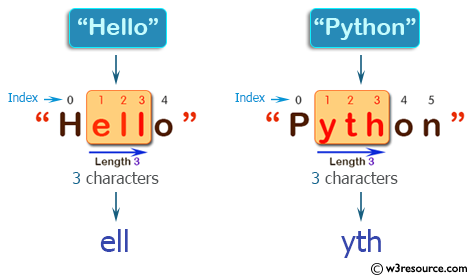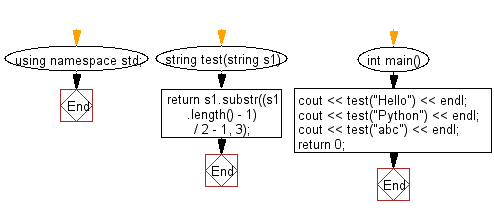﻿ C++ : New string from 3 characters from middle of a string# C++ Exercises: Create a new string taking 3 characters from the middle of a given string at least 3

## C++ Basic Algorithm: Exercise-70 with Solution

Write a C++ program to create a new string taking 3 characters from the middle of a given string at least 3.

Sample Solution:

C++ Code :

``````#include <iostream>
using namespace std;

string test(string s1)
{
return s1.substr((s1.length() - 1) / 2 - 1, 3);
}

int main()
{
cout << test("Hello") << endl;
cout << test("Python") << endl;
cout << test("abc") << endl;
return 0;
}
``````

Sample Output:

```ell
yth
abc
```

Pictorial Presentation:Flowchart:C++ Code Editor: